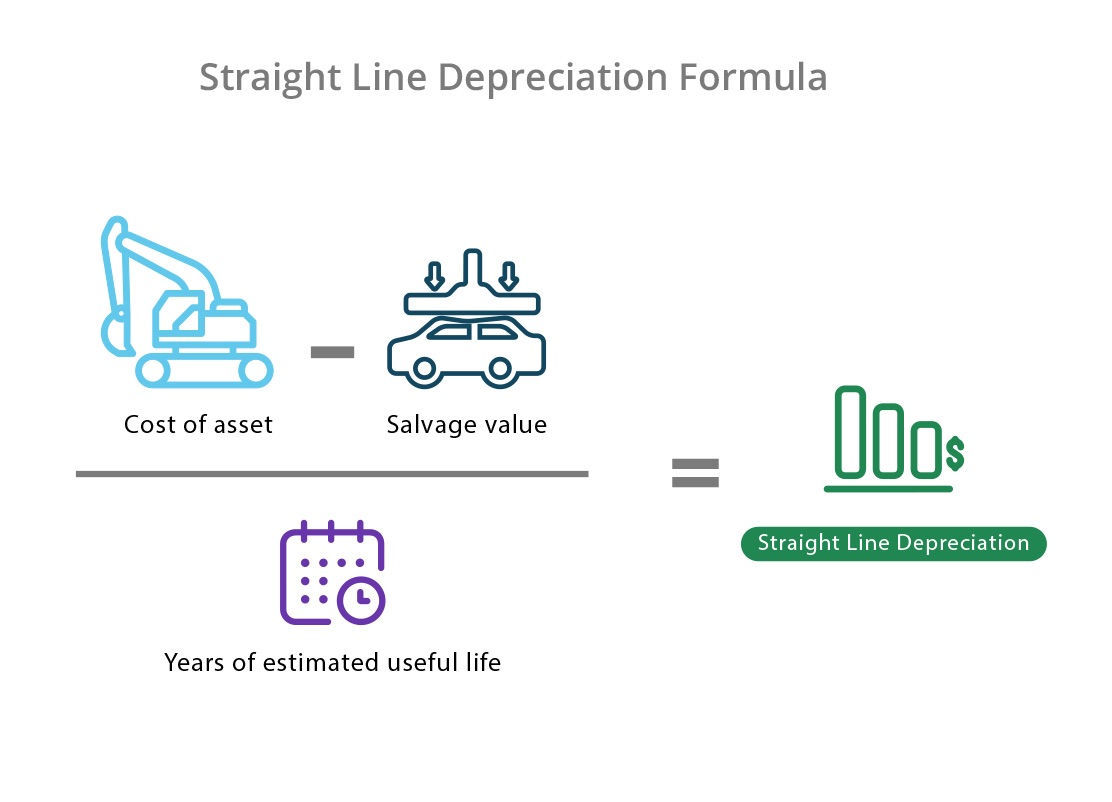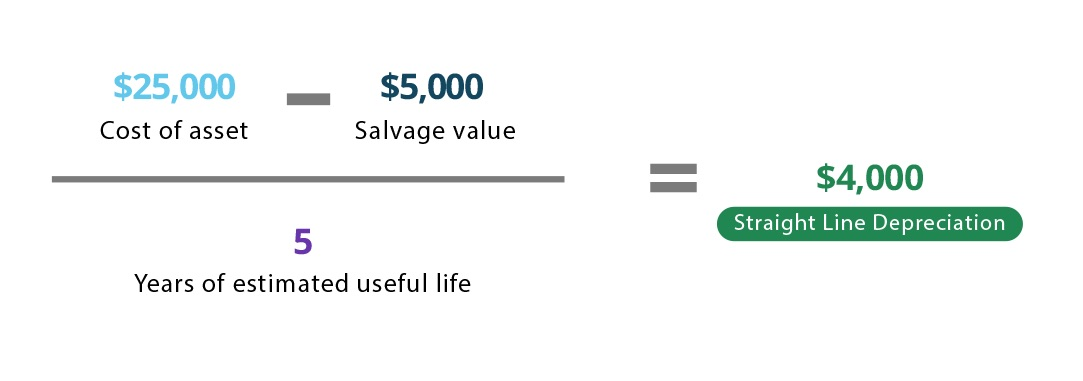# Depreciation

Updated June 30, 2021

## What Is Depreciation?

Depreciation in accounting is a method that measures the reduction in an asset’s value over the course of its useful life. It also represents how much of an asset’s value is depleted due to usage, wear and tear, or obsolescence.

## Why Is Depreciation Important?

Depreciation allows businesses to track the cost of assets for accounting, tax, and operational purposes.

When a company buys a fixed asset (such as a vehicle or manufacturing equipment), that asset loses substantial value over time. Rather than record a single large expense for the reduction in value within the first year, accountants typically depreciate the asset and spread out its expense over several years.

This allows companies to approximate the useful life of an asset in order to match the benefit derived from its usage. Depreciation expenses can also provide tax deductions and reduce taxable earnings to decrease the amount companies pay in taxes. The choice of accounting method can affect the way depreciation is recorded:

## Where Do I Find Depreciation?

Depreciation expenses appear on a profit and loss statement. Book value and accumulated depreciation accounts, however, appear on a balance sheet.

## Formulas & Examples of Depreciation

Since there are so many types of depreciation, there isn’t a single depreciation formula. The following section outlines the different methods and formulas by using simple depreciation examples.

### 1. Straight Line Depreciation

The most straightforward method, straight line depreciation is most often used when the value of an asset decreases evenly (rather than gradually) over its useful life. On balance sheets, businesses will report an equal depreciation cost every year until the asset reaches its salvage value.

#### Straight Line Depreciation Formula#### Straight Line Depreciation Example

Company ABC buys a tractor for \$25,000 and expects it to last four years (at which point it will be salvaged for \$5,000).End of YearBook valueDepreciation expenseAccumulated Depreciation
0\$25,000\$0\$0
1\$20,000\$5,000\$5,000
2\$15,000\$5,000\$10,000
3\$10,000\$5,000\$15,000
4\$5,000\$5,000\$20,000

Note: The depreciation expense appears on a profit and loss statement, while the book value and accumulated depreciation accounts appear on a balance sheet.

Using the straight line depreciation method, the tractor would depreciate by \$5,000 per year for a total accumulated depreciation of \$20,000. Once the book value equals the original salvage value, it is considered a fully-depreciated asset.

The company’s accountants would record a non-cash expense of \$5,000 on the profit and loss statement each year until the end of the tractor’s useful life (in this example, four years).

Note: Since the business already spent cash when the asset was purchased, depreciation is considered a non-cash expense.

### 2. Declining Balance Depreciation

Declining balance depreciation is a type of accelerated depreciation that allows companies to account for greater depreciation in earlier years.  The purchase of a vehicle is a good example, since vehicles generally lose a large portion of their value within the first year.

Instead of evenly distributing the cost of depreciation over the course of an asset’s useful life, this method depreciates the book value of the asset by the straight line depreciation percent each year.

Since the depreciation expense doesn’t apply until the end of the year, the book value at the beginning of year one would simply be the cost of the asset. The book value for each following year would be the previous year’s book value minus the cost of depreciation. And because the value of the asset is highest in the earlier years, there is a larger initial depreciation cost that declines over time.

#### Declining Balance Depreciation Formula

(Cost - accumulated depreciation)

Declining balance depreciation = Book value * Straight line depreciation percent

*Where straight line depreciation percent =  1 / useful life of asset (in years)

#### Declining Balance Depreciation Example

Company XYZ buys a tractor for \$25,000 and expects it to last four years, at which point it will be salvaged for \$5,000. The book value in this situation would be \$25,000 and the straight line depreciation percent would be 25% (1 / 4 years). Here’s how the tractor would depreciate over the four years:

Book valueDepreciation expenseAccumulated Depreciation
End of yearBook valueDepreciation expenseAccumulated depreciation
0\$25,000\$0\$0
1\$18,750\$6,250\$6,250
2\$14,062\$4,688\$10,938
3\$10,546\$3,516\$14,454
4\$5,000\$5,546\$20,000

### 3. Double-Declining Balance Depreciation

Double-declining balance depreciation is a more rapid form of accelerated depreciation. It works just like the previous method but doubles the straight line depreciation percent, so it’s best suited for assets that lose value rapidly.

#### Double-Declining Balance Depreciation Formula

Declining balance depreciation = Book value * (Straight line depreciation percent x 2)

#### Double-Declining Balance Depreciation Example

Company XYZ buys a tractor for \$25,000 and expects it to last four years, at which point it will be salvaged for \$5,000.

The book value in this situation would be \$25,000. The straight line depreciation percent would be 25% (1 / 4 years), meaning the double-declining depreciation rate would be 50%. Here’s how the tractor would depreciate over the five years:

Book valueDepreciation expenseAccumulated Depreciation
End of yearBook valueDepreciation expenseAccumulated depreciation
0\$25,000\$0\$0
1\$12,500\$12,500\$12,500
2\$5,000\$7,500\$20,000
3\$5,000\$0\$20,000
4\$5,000\$0\$20,000

### 4. 150% Declining Balance Depreciation

The 150% declining balance depreciation method is a slightly slower version of the double declining balance method. Instead of using twice the straight-line value, it uses 150% (or 1.5x).

#### 150% Declining Balance Depreciation Formula

Declining balance depreciation = Book value * (Straight line depreciation percent x 1.5)

#### 150% Declining Balance Example

Company XYZ buys a tractor for \$25,000 and expects it to last four years, at which point it would be salvaged for \$5,000. The book value in this situation would be \$25,000. The straight line depreciation percent would be 25% (1 / 4 years), meaning the 150% declining balance depreciation rate would be 37.5%. Here’s how the tractor would depreciate over the four years:

Book valueDepreciation expenseAccumulated Depreciation
End of yearBook valueDepreciation expenseAccumulated depreciation
0\$25,000\$0\$0
1\$15,625\$9,375\$9,375
2\$9,766\$5,859\$15,234
3\$6,104\$3,662\$18,896
4\$5,000\$1,104\$20,000

### 5. Modified Accelerated Cost Recovery System (MACRS) Depreciation

Modified Accelerated Cost Recovery System (or MACRS) is the current US tax depreciation system that companies are required to use when depreciating their assets. Under this system, companies can recover the capitalized cost of an asset through annual deductions. MACRS is an accelerated depreciation system that depreciates most of an asset’s value in the first few years.

There are two systems under MACRS:

• GDS (General Depreciation System) - Most common and uses the declining balance method.
• ADS (Alternative Depreciation System) - Depreciates an asset’s value over a longer period of time and has smaller yearly deductions.

To depreciate an asset using MACRS, companies must first determine the asset’s classification and which system to use. Once that has been determined, the company must consult IRS Pub 946 to identify the correct depreciation method and depreciation rate for the asset.

#### Modified Accelerated Cost Recovery System (MACRS) Example

Company XYZ buys office furniture (considered a 7-year property) for \$10,000. According to the IRS table A-1, 7-year property is subject to 14.29% depreciation in the first year.

In this situation, the depreciation expense for year 1 would be: \$10,000 * 14.29% = \$1,429. The book value would be: \$10,000 - \$1,429 = \$8,571.

To calculate depreciation for each following year, the company would multiply the book value (cost - MARC depreciation % for that year) of the office furniture by the corresponding depreciation rate for that year.

Note: This is a simple example of MACRS. There are often multiple variables that can affect depreciation, like the date an asset is placed in service, the business use of property, and disposal date.

### Units of Production Depreciation Method

Some manufacturing companies use the units of production depreciation method to calculate the useful life of equipment. Rather than using time, this method measures an asset's value with respect to the number of units it's expected to produce over the course of its useful life.

#### Units of Production Depreciation Method Example

Company XYZ has a machine that is expected to produce 500,000 hats over its lifetime. If it produces 100,000 hats, it will have used up 20% of its value (100,000 completed units / 500,000 expected units).

## Why Is Depreciation Important?

Depreciation allows businesses to track the cost of assets for accounting, tax, and operational purposes.

When a company buys a fixed asset (such as a vehicle or manufacturing equipment), that asset loses substantial value over time. Rather than record a single large expense for the reduction in value within the first year, accountants typically depreciate the asset and spread out its expense over several years.

This allows companies to approximate the useful life of an asset in order to match the benefit derived from its usage. Depreciation expenses can also provide tax deductions and reduce taxable earnings to decrease the amount companies pay in taxes. The choice of accounting method can affect the way depreciation is recorded:

All of our content is verified for accuracy by Victoria Chan, CFA and our team of certified financial experts. We pride ourselves on quality, research, and transparency, and we value your feedback. Below you'll find answers to some of the most common reader questions about Depreciation.
##### Be the first to ask a question

Victoria Chan, CFA - 153Victoria Chan, CFACFA Charterholder

Chartered Financial Analyst

Vicky has over 10 years' experience in financial services, working in strategy and management consulting. She has also served as the deputy head of research for citibank's consumer bank in Australia, advising financial planners and clients on investment strategies and asset allocation.